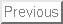## Exam Given November 5, 2002

1. Planes:

a) Find the equation of the plane that goes through the point (2,1,0) and has normal vector (2,1,-2).

Let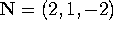. The equation of the plane is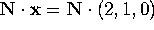, or 2 x + y -2 z =5.

b) Find the distance from the point (4,13,5) to the plane in part (a).

The distance is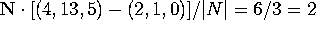.

c) Find the equation of the plane that goes through the three points (2,1,0), (3,1,4) and (0,0,0) [No, this isn't the same plane as part a)].

Since one of the points is the origin, the normal vector to this plane is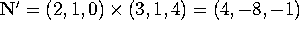, so the plane is 4x - 8y - z =0.

d) Find the cosine of the angle between the planes of part (a) and (c).

This is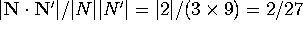.

2. Lines

a) Find the equation, in symmetric form, for the line through the point (2,1,0) in the direction (2,1,-2).

The direction vector is d=(2,1,-2), the base point is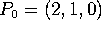, so the line is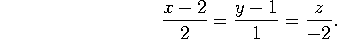b) How far is the origin (0,0,0) from this line?

The vector from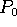to the origin is v=(-2,-1,0). The distance is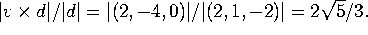c) Find the equation, in symmetric form, for the line through the two points (2,1,0) and (3,-1,4).

The direction vector is d' = (3,-1,4)-(2,1,0) = (1,-2,4), so our line is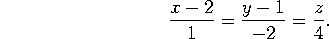You could also write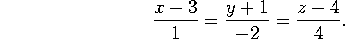d) Find the equation (in symmetric form) of the line through (2,1,0) that is perpendicular to the lines of parts (a) and (c).

The vector normal to d and d' is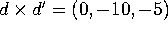. Rescale it to (0,2,1). Our line is(If you don't rescale the direction vector, then you get an uglier, but completely equivalent, answer.)

3. Parametrized curves: Consider the parametrized curve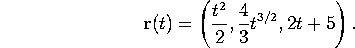a) Compute the position, velocity, unit tangent vector and speed at time t=1.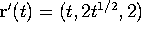, so position =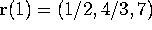, velocity =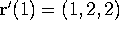, speed = |(1,2,2)| = 3, and unit tangent vector =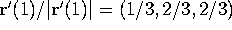.

b) Compute the arc-length of the curve from t=0 to t=2.

Note that the speed is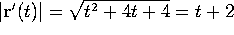, so the arclength is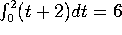.

4. Polar coordinates.

a) Sketch the curve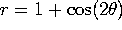. Mark clearly the angles (if any) where the curve goes through the origin, and the angles where r is maximal.

There are two lobes, one to the right and one to the left. The curve goes through the origin at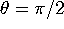and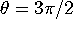. The farthest points are when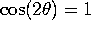, so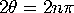, so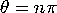. That is, the positive and negative x directions.

b) Find (in polar coordinates!) the points where this curve intersects the circle r=1/2.

Note that r is never negative, so we don't have to worry about ``accidental'' intersections. Set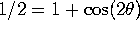, so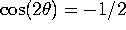, to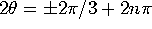, so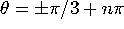. For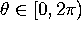, these are the points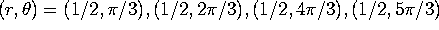.

c) Write down a definite integral that gives the area, in the first quadrant, inside the curve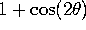but outside the circle r=1/2.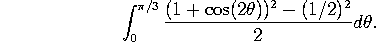Extra credit: Evaluate this integral. Expand it out and use the double-angle formula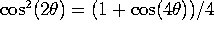to convert the integral to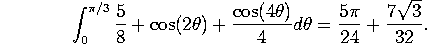5. Partial derivatives. Consider the function of two variables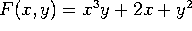.

a) Compute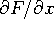and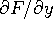and evaluate these at the point (1,1).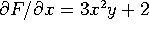, evaluated at (1,1) gives 5.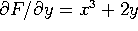, evaluated at (1,1) gives 3.

b) Use these to estimate the value of F(1.01, 1) and the value of F(1, 1.01).

The change in F from (1,1) to (1.01,1) is roughly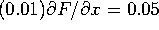, so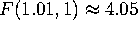. The change in F from (1,1) to (1,1.01) is roughly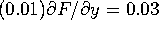, so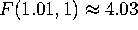.

c) Estimate the value of F(1.02, 1.01).

Add the effect of changing x to the effect of changing y: 4 + 5(0.02) + 3(0.01) = 4.13.

d) Compute the second-order partial derivatives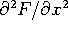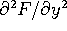, and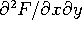.

In order, the answers are 6xy, 2, and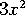.